# Medical Expenditure Tutorial¶

## This tutorial demonstrates classification model learning with bias mitigation as a part of a Care Management use case using Medical Expenditure data.¶

The notebook demonstrates how the AIF 360 toolkit can be used to detect and reduce bias when learning classifiers using a variety of fairness metrics and algorithms . It also demonstrates how explanations can be generated for predictions made by models learnt with the toolkit using LIME.

Classifiers are built using Logistic Regression as well as Random Forests.

Bias detection is demonstrated using several metrics, including disparate impact, average odds difference, statistical parity difference, equal opportunity difference, and Theil index.

Bias alleviation is explored via a variety of methods, including reweighing (pre-processing algorithm), prejudice remover (in-processing algorithm), and disparate impact remover (pre-processing technique).

Data from the Medical Expenditure Panel Survey is used in this tutorial. See Section 2 below for more details.

## 1. Use case¶

In order to demonstrate how AIF 360 can be used to detect and mitigate bias in classfier models, we adopt the following use case:

1. a data scientist develops a 'fair' healthcare utilization scoring model with respect to defined protected classes. Fairness may be dictated by legal or government regulations, such as a requirement that additional care decisions be not predicated on factors such as race of the patient.
1. developer takes the model AND performance characteristics / specs of the model (e.g. accuracy, fairness tests, etc. basically the model factsheet) and deploys the model in an enterprise app that prioritizes cases for care management.
1. the app is put into production and starts scoring people and making recommendations.
1. explanations are generated for each recommendation
1. both recommendations and associated explanations are given to nurses as a part of the care management process. The nurses can evaluate the recommendations for quality and correctness and provide feedback.
1. nurse feedback as well as analysis of usage data with respect to specs of the model w.r.t accuracy and fairness is communicated to AI Ops specialist and LOB user periodically.
1. when significant drift in model specs relative to the model factsheet is observed, the model is sent back for retraining.

## 2. Data used¶

The 2015 file contains data from rounds 3,4,5 of panel 19 (2014) and rounds 1,2,3 of panel 20 (2015). The 2016 file contains data from rounds 3,4,5 of panel 20 (2015) and rounds 1,2,3 of panel 21 (2016).

For this demonstration, three datasets were constructed: one from panel 19, round 5 (used for learning models), one from panel 20, round 3 (used for deployment/testing of model - steps); the other from panel 21, round 3 (used for re-training and deployment/testing of updated model).

For each dataset, the sensitive attribute is 'RACE' constructed as follows: 'Whites' (privileged class) defined by the features RACEV2X = 1 (White) and HISPANX = 2 (non Hispanic); 'Non-Whites' that included everyone else.

Along with race as the sensitive feature, other features used for modeling include demographics (such as age, gender, active duty status), physical/mental health assessments, diagnosis codes (such as history of diagnosis of cancer, or diabetes), and limitations (such as cognitive or hearing or vision limitation).

To measure utilization, a composite feature, 'UTILIZATION', was created to measure the total number of trips requiring some sort of medical care by summing up the following features: OBTOTV15(16), the number of office based visits; OPTOTV15(16), the number of outpatient visits; ERTOT15(16), the number of ER visits; IPNGTD15(16), the number of inpatient nights, and + HHTOTD16, the number of home health visits.

The model classification task is to predict whether a person would have 'high' utilization (defined as UTILIZATION >= 10, roughly the average utilization for the considered population). High utilization respondents constituted around 17% of each dataset.

To simulate the scenario, each dataset is split into 3 parts: a train, a validation, and a test/deployment part.

We assume that the model is initially built and tuned using the 2015 Panel 19 train/test data. (Use case steps 1-2.) It is then put into practice and used to score people to identify potential candidates for care management (Use case steps 3-5). Initial deployment is simulated to 2015 Panel 20 deployment data. To show change in performance and/or fairness over time, (use case steps 6-7), the 2016 Panel 21 deployment data is used. Finally, if drift is observed, the 2015 train/validation data is used to learn a new model and evaluated again on the 2016 deployment data

## 3. Training models on original 2015 Panel 19 data¶

In :
import sys
sys.path.insert(0, '../')

%matplotlib inline
import matplotlib.pyplot as plt
import numpy as np
from IPython.display import Markdown, display

# Datasets
from aif360.datasets import MEPSDataset19
from aif360.datasets import MEPSDataset20
from aif360.datasets import MEPSDataset21

# Fairness metrics
from aif360.metrics import BinaryLabelDatasetMetric
from aif360.metrics import ClassificationMetric

# Explainers
from aif360.explainers import MetricTextExplainer

# Scalers
from sklearn.preprocessing import StandardScaler

# Classifiers
from sklearn.ensemble import RandomForestClassifier
from sklearn.linear_model import LogisticRegression
from sklearn.pipeline import make_pipeline

# Bias mitigation techniques
from aif360.algorithms.preprocessing import Reweighing
from aif360.algorithms.inprocessing import PrejudiceRemover

# LIME
from aif360.datasets.lime_encoder import LimeEncoder
import lime
from lime.lime_tabular import LimeTabularExplainer

np.random.seed(1)


### 3.1. Load data & create splits for learning/validating/testing model¶

Get the dataset and split into train (50%), validate (30%), and test (20%)

In :
(dataset_orig_panel19_train,
dataset_orig_panel19_val,
dataset_orig_panel19_test) = MEPSDataset19().split([0.5, 0.8], shuffle=True)

sens_ind = 0
sens_attr = dataset_orig_panel19_train.protected_attribute_names[sens_ind]

unprivileged_groups = [{sens_attr: v} for v in
dataset_orig_panel19_train.unprivileged_protected_attributes[sens_ind]]
privileged_groups = [{sens_attr: v} for v in
dataset_orig_panel19_train.privileged_protected_attributes[sens_ind]]


This function will be used throughout the notebook to print out some labels, names, etc.

In :
def describe(train=None, val=None, test=None):
if train is not None:
display(Markdown("#### Training Dataset shape"))
print(train.features.shape)
if val is not None:
display(Markdown("#### Validation Dataset shape"))
print(val.features.shape)
display(Markdown("#### Test Dataset shape"))
print(test.features.shape)
display(Markdown("#### Favorable and unfavorable labels"))
print(test.favorable_label, test.unfavorable_label)
display(Markdown("#### Protected attribute names"))
print(test.protected_attribute_names)
display(Markdown("#### Privileged and unprivileged protected attribute values"))
print(test.privileged_protected_attributes,
test.unprivileged_protected_attributes)
display(Markdown("#### Dataset feature names"))
print(test.feature_names)


Show 2015 dataset details

In :
describe(dataset_orig_panel19_train, dataset_orig_panel19_val, dataset_orig_panel19_test)


#### Training Dataset shape¶

(7915, 138)


#### Validation Dataset shape¶

(4749, 138)


#### Test Dataset shape¶

(3166, 138)


#### Favorable and unfavorable labels¶

1.0 0.0


#### Protected attribute names¶

['RACE']


#### Privileged and unprivileged protected attribute values¶

[array([1.])] [array([0.])]


#### Dataset feature names¶

['AGE', 'RACE', 'PCS42', 'MCS42', 'K6SUM42', 'REGION=1', 'REGION=2', 'REGION=3', 'REGION=4', 'SEX=1', 'SEX=2', 'MARRY=1', 'MARRY=2', 'MARRY=3', 'MARRY=4', 'MARRY=5', 'MARRY=6', 'MARRY=7', 'MARRY=8', 'MARRY=9', 'MARRY=10', 'FTSTU=-1', 'FTSTU=1', 'FTSTU=2', 'FTSTU=3', 'ACTDTY=1', 'ACTDTY=2', 'ACTDTY=3', 'ACTDTY=4', 'HONRDC=1', 'HONRDC=2', 'HONRDC=3', 'HONRDC=4', 'RTHLTH=-1', 'RTHLTH=1', 'RTHLTH=2', 'RTHLTH=3', 'RTHLTH=4', 'RTHLTH=5', 'MNHLTH=-1', 'MNHLTH=1', 'MNHLTH=2', 'MNHLTH=3', 'MNHLTH=4', 'MNHLTH=5', 'HIBPDX=-1', 'HIBPDX=1', 'HIBPDX=2', 'CHDDX=-1', 'CHDDX=1', 'CHDDX=2', 'ANGIDX=-1', 'ANGIDX=1', 'ANGIDX=2', 'MIDX=-1', 'MIDX=1', 'MIDX=2', 'OHRTDX=-1', 'OHRTDX=1', 'OHRTDX=2', 'STRKDX=-1', 'STRKDX=1', 'STRKDX=2', 'EMPHDX=-1', 'EMPHDX=1', 'EMPHDX=2', 'CHBRON=-1', 'CHBRON=1', 'CHBRON=2', 'CHOLDX=-1', 'CHOLDX=1', 'CHOLDX=2', 'CANCERDX=-1', 'CANCERDX=1', 'CANCERDX=2', 'DIABDX=-1', 'DIABDX=1', 'DIABDX=2', 'JTPAIN=-1', 'JTPAIN=1', 'JTPAIN=2', 'ARTHDX=-1', 'ARTHDX=1', 'ARTHDX=2', 'ARTHTYPE=-1', 'ARTHTYPE=1', 'ARTHTYPE=2', 'ARTHTYPE=3', 'ASTHDX=1', 'ASTHDX=2', 'ADHDADDX=-1', 'ADHDADDX=1', 'ADHDADDX=2', 'PREGNT=-1', 'PREGNT=1', 'PREGNT=2', 'WLKLIM=-1', 'WLKLIM=1', 'WLKLIM=2', 'ACTLIM=-1', 'ACTLIM=1', 'ACTLIM=2', 'SOCLIM=-1', 'SOCLIM=1', 'SOCLIM=2', 'COGLIM=-1', 'COGLIM=1', 'COGLIM=2', 'DFHEAR42=-1', 'DFHEAR42=1', 'DFHEAR42=2', 'DFSEE42=-1', 'DFSEE42=1', 'DFSEE42=2', 'ADSMOK42=-1', 'ADSMOK42=1', 'ADSMOK42=2', 'PHQ242=-1', 'PHQ242=0', 'PHQ242=1', 'PHQ242=2', 'PHQ242=3', 'PHQ242=4', 'PHQ242=5', 'PHQ242=6', 'EMPST=-1', 'EMPST=1', 'EMPST=2', 'EMPST=3', 'EMPST=4', 'POVCAT=1', 'POVCAT=2', 'POVCAT=3', 'POVCAT=4', 'POVCAT=5', 'INSCOV=1', 'INSCOV=2', 'INSCOV=3']


Metrics for original data

In :
metric_orig_panel19_train = BinaryLabelDatasetMetric(
dataset_orig_panel19_train,
unprivileged_groups=unprivileged_groups,
privileged_groups=privileged_groups)
explainer_orig_panel19_train = MetricTextExplainer(metric_orig_panel19_train)

print(explainer_orig_panel19_train.disparate_impact())

Disparate impact (probability of favorable outcome for unprivileged instances / probability of favorable outcome for privileged instances): 0.48230522996275893


### 3.2. Learning a Logistic Regression (LR) classifier on original data¶

#### 3.2.1. Training LR model on original data¶

In :
dataset = dataset_orig_panel19_train
model = make_pipeline(StandardScaler(),
LogisticRegression(solver='liblinear', random_state=1))
fit_params = {'logisticregression__sample_weight': dataset.instance_weights}

lr_orig_panel19 = model.fit(dataset.features, dataset.labels.ravel(), **fit_params)


#### 3.2.2. Validating LR model on original data¶

This function will be used throughout the tutorial to find best threshold using a validation set

In :
from collections import defaultdict

def test(dataset, model, thresh_arr):
try:
# sklearn classifier
y_val_pred_prob = model.predict_proba(dataset.features)
pos_ind = np.where(model.classes_ == dataset.favorable_label)
except AttributeError:
# aif360 inprocessing algorithm
y_val_pred_prob = model.predict(dataset).scores
pos_ind = 0

metric_arrs = defaultdict(list)
for thresh in thresh_arr:
y_val_pred = (y_val_pred_prob[:, pos_ind] > thresh).astype(np.float64)

dataset_pred = dataset.copy()
dataset_pred.labels = y_val_pred
metric = ClassificationMetric(
dataset, dataset_pred,
unprivileged_groups=unprivileged_groups,
privileged_groups=privileged_groups)

metric_arrs['bal_acc'].append((metric.true_positive_rate()
+ metric.true_negative_rate()) / 2)
metric_arrs['avg_odds_diff'].append(metric.average_odds_difference())
metric_arrs['disp_imp'].append(metric.disparate_impact())
metric_arrs['stat_par_diff'].append(metric.statistical_parity_difference())
metric_arrs['eq_opp_diff'].append(metric.equal_opportunity_difference())
metric_arrs['theil_ind'].append(metric.theil_index())

return metric_arrs

In :
thresh_arr = np.linspace(0.01, 0.5, 50)
val_metrics = test(dataset=dataset_orig_panel19_val,
model=lr_orig_panel19,
thresh_arr=thresh_arr)
lr_orig_best_ind = np.argmax(val_metrics['bal_acc'])


Plot metrics with twin x-axes

In :
def plot(x, x_name, y_left, y_left_name, y_right, y_right_name):
fig, ax1 = plt.subplots(figsize=(10,7))
ax1.plot(x, y_left)
ax1.set_xlabel(x_name, fontsize=16, fontweight='bold')
ax1.set_ylabel(y_left_name, color='b', fontsize=16, fontweight='bold')
ax1.xaxis.set_tick_params(labelsize=14)
ax1.yaxis.set_tick_params(labelsize=14)
ax1.set_ylim(0.5, 0.8)

ax2 = ax1.twinx()
ax2.plot(x, y_right, color='r')
ax2.set_ylabel(y_right_name, color='r', fontsize=16, fontweight='bold')
if 'disparate impact' in y_right_name:
ax2.set_ylim(0., 0.7)
else:
ax2.set_ylim(-0.25, 0.1)

best_ind = np.argmax(y_left)
ax2.axvline(np.array(x)[best_ind], color='k', linestyle=':')
ax2.yaxis.set_tick_params(labelsize=14)
ax2.grid(True)


Here we plot $1 - \min(\text{disparate impact}, 1/\text{disparate impact})$ since it's possible to overcorrect and end up with a value greater than 1, implying unfairness for the original privileged group. For shorthand, we simply call this 1-min(DI, 1/DI) from now on. We want the plotted metric to be less than 0.2.

In :
disp_imp = np.array(val_metrics['disp_imp'])
disp_imp_err = 1 - np.minimum(disp_imp, 1/disp_imp)
plot(thresh_arr, 'Classification Thresholds',
val_metrics['bal_acc'], 'Balanced Accuracy',
disp_imp_err, '1 - min(DI, 1/DI)')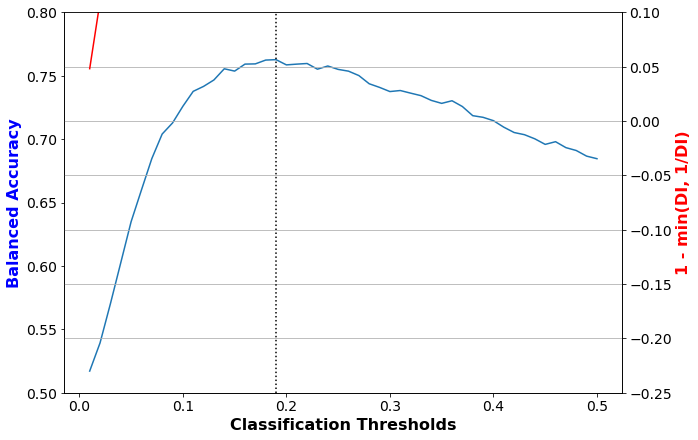In :
plot(thresh_arr, 'Classification Thresholds',
val_metrics['bal_acc'], 'Balanced Accuracy',
val_metrics['avg_odds_diff'], 'avg. odds diff.')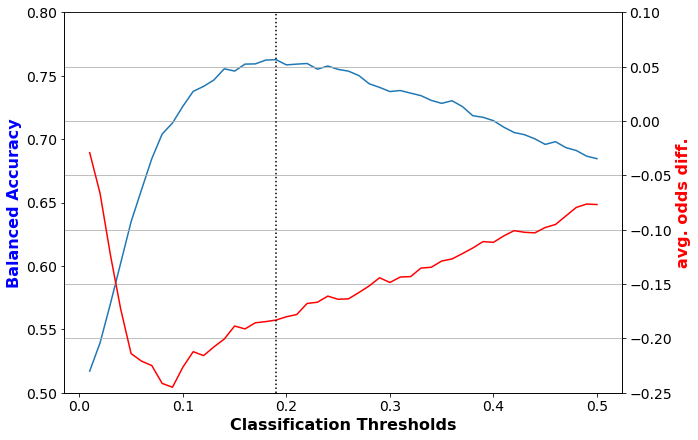Make a function to print out accuracy and fairness metrics. This will be used throughout the tutorial.

In :
def describe_metrics(metrics, thresh_arr):
best_ind = np.argmax(metrics['bal_acc'])
print("Threshold corresponding to Best balanced accuracy: {:6.4f}".format(thresh_arr[best_ind]))
print("Best balanced accuracy: {:6.4f}".format(metrics['bal_acc'][best_ind]))
#     disp_imp_at_best_ind = np.abs(1 - np.array(metrics['disp_imp']))[best_ind]
disp_imp_at_best_ind = 1 - min(metrics['disp_imp'][best_ind], 1/metrics['disp_imp'][best_ind])
print("Corresponding 1-min(DI, 1/DI) value: {:6.4f}".format(disp_imp_at_best_ind))
print("Corresponding average odds difference value: {:6.4f}".format(metrics['avg_odds_diff'][best_ind]))
print("Corresponding statistical parity difference value: {:6.4f}".format(metrics['stat_par_diff'][best_ind]))
print("Corresponding equal opportunity difference value: {:6.4f}".format(metrics['eq_opp_diff'][best_ind]))
print("Corresponding Theil index value: {:6.4f}".format(metrics['theil_ind'][best_ind]))

In :
describe_metrics(val_metrics, thresh_arr)

Threshold corresponding to Best balanced accuracy: 0.1900
Best balanced accuracy: 0.7627
Corresponding 1-min(DI, 1/DI) value: 0.6066
Corresponding average odds difference value: -0.1831
Corresponding statistical parity difference value: -0.2643
Corresponding equal opportunity difference value: -0.1608
Corresponding Theil index value: 0.0936


#### 3.2.3. Testing LR model on original data¶

In :
lr_orig_metrics = test(dataset=dataset_orig_panel19_test,
model=lr_orig_panel19,
thresh_arr=[thresh_arr[lr_orig_best_ind]])

In :
describe_metrics(lr_orig_metrics, [thresh_arr[lr_orig_best_ind]])

Threshold corresponding to Best balanced accuracy: 0.1900
Best balanced accuracy: 0.7759
Corresponding 1-min(DI, 1/DI) value: 0.5738
Corresponding average odds difference value: -0.2057
Corresponding statistical parity difference value: -0.2612
Corresponding equal opportunity difference value: -0.2228
Corresponding Theil index value: 0.0921


For all the fairness metrics displayed above, the value should be close to '0' for fairness.

1-min(DI, 1/DI) < 0.2 is typically desired for classifier predictions to be fair.

However, for a logistic regression classifier trained with original training data, at the best classification rate, this is quite high. This implies unfairness.

Similarly, $\text{average odds difference} = \frac{(FPR_{unpriv}-FPR_{priv})+(TPR_{unpriv}-TPR_{priv})}{2}$ must be close to zero for the classifier to be fair.

Again, the results for this classifier-data combination are still high. This still implies unfairness.

### 3.3. Learning a Random Forest (RF) classifier on original data¶

#### 3.3.1. Training RF model on original data¶

In :
dataset = dataset_orig_panel19_train
model = make_pipeline(StandardScaler(),
RandomForestClassifier(n_estimators=500, min_samples_leaf=25))
fit_params = {'randomforestclassifier__sample_weight': dataset.instance_weights}
rf_orig_panel19 = model.fit(dataset.features, dataset.labels.ravel(), **fit_params)


#### 3.3.2. Validating RF model on original data¶

In :
thresh_arr = np.linspace(0.01, 0.5, 50)
val_metrics = test(dataset=dataset_orig_panel19_val,
model=rf_orig_panel19,
thresh_arr=thresh_arr)
rf_orig_best_ind = np.argmax(val_metrics['bal_acc'])

In :
disp_imp = np.array(val_metrics['disp_imp'])
disp_imp_err = 1 - np.minimum(disp_imp, 1/disp_imp)
plot(thresh_arr, 'Classification Thresholds',
val_metrics['bal_acc'], 'Balanced Accuracy',
disp_imp_err, '1 - min(DI, 1/DI)')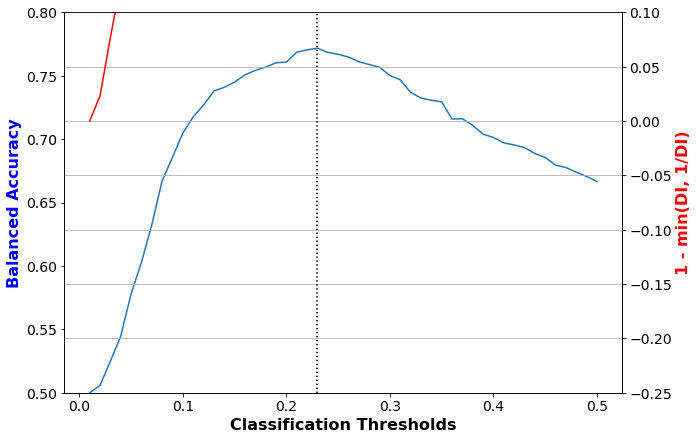In :
plot(thresh_arr, 'Classification Thresholds',
val_metrics['bal_acc'], 'Balanced Accuracy',
val_metrics['avg_odds_diff'], 'avg. odds diff.')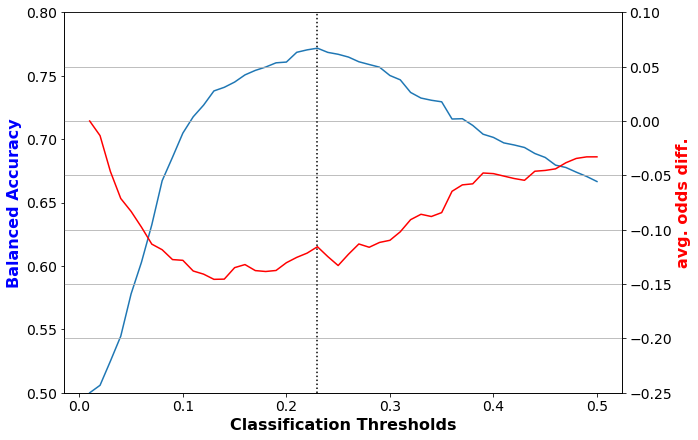In :
describe_metrics(val_metrics, thresh_arr)

Threshold corresponding to Best balanced accuracy: 0.2300
Best balanced accuracy: 0.7717
Corresponding 1-min(DI, 1/DI) value: 0.4860
Corresponding average odds difference value: -0.1157
Corresponding statistical parity difference value: -0.1929
Corresponding equal opportunity difference value: -0.1063
Corresponding Theil index value: 0.0896


#### 3.3.3. Testing RF model on original data¶

In :
rf_orig_metrics = test(dataset=dataset_orig_panel19_test,
model=rf_orig_panel19,
thresh_arr=[thresh_arr[rf_orig_best_ind]])

In :
describe_metrics(rf_orig_metrics, [thresh_arr[rf_orig_best_ind]])

Threshold corresponding to Best balanced accuracy: 0.2300
Best balanced accuracy: 0.7638
Corresponding 1-min(DI, 1/DI) value: 0.5141
Corresponding average odds difference value: -0.1388
Corresponding statistical parity difference value: -0.2190
Corresponding equal opportunity difference value: -0.1135
Corresponding Theil index value: 0.0936


As in the case of the logistic regression classifier learned on the original data, the fairness metrics for the random forest classifier have values that are quite far from 0.

For example, 1 - min(DI, 1/DI) has a value of over 0.5 as opposed to the desired value of < 0.2.

This indicates that the random forest classifier learned on the original data is also unfair.

## 4. Bias mitigation using pre-processing technique - Reweighing¶

### 4.1. Transform data¶

In :
RW = Reweighing(unprivileged_groups=unprivileged_groups,
privileged_groups=privileged_groups)
dataset_transf_panel19_train = RW.fit_transform(dataset_orig_panel19_train)


Metrics for transformed data

In :
metric_transf_panel19_train = BinaryLabelDatasetMetric(
dataset_transf_panel19_train,
unprivileged_groups=unprivileged_groups,
privileged_groups=privileged_groups)
explainer_transf_panel19_train = MetricTextExplainer(metric_transf_panel19_train)

print(explainer_transf_panel19_train.disparate_impact())

Disparate impact (probability of favorable outcome for unprivileged instances / probability of favorable outcome for privileged instances): 1.0000000000000002


### 4.2. Learning a Logistic Regression (LR) classifier on data transformed by reweighing¶

#### 4.2.1. Training LR model after reweighing¶

In :
dataset = dataset_transf_panel19_train
model = make_pipeline(StandardScaler(),
LogisticRegression(solver='liblinear', random_state=1))
fit_params = {'logisticregression__sample_weight': dataset.instance_weights}
lr_transf_panel19 = model.fit(dataset.features, dataset.labels.ravel(), **fit_params)


#### 4.2.2. Validating LR model after reweighing¶

In :
thresh_arr = np.linspace(0.01, 0.5, 50)
val_metrics = test(dataset=dataset_orig_panel19_val,
model=lr_transf_panel19,
thresh_arr=thresh_arr)
lr_transf_best_ind = np.argmax(val_metrics['bal_acc'])

In :
disp_imp = np.array(val_metrics['disp_imp'])
disp_imp_err = 1 - np.minimum(disp_imp, 1/disp_imp)
plot(thresh_arr, 'Classification Thresholds',
val_metrics['bal_acc'], 'Balanced Accuracy',
disp_imp_err, '1 - min(DI, 1/DI)')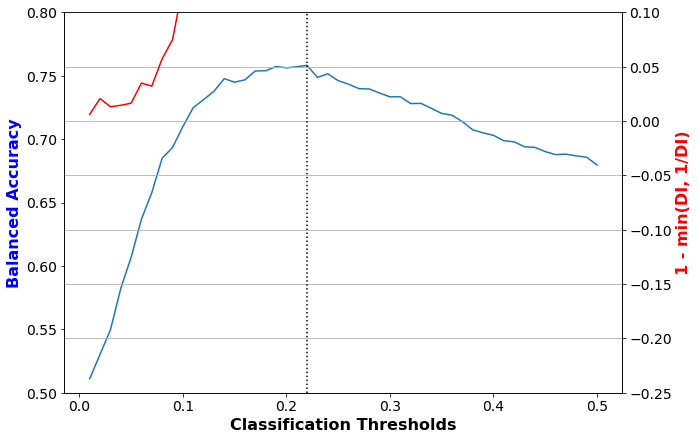In :
plot(thresh_arr, 'Classification Thresholds',
val_metrics['bal_acc'], 'Balanced Accuracy',
val_metrics['avg_odds_diff'], 'avg. odds diff.')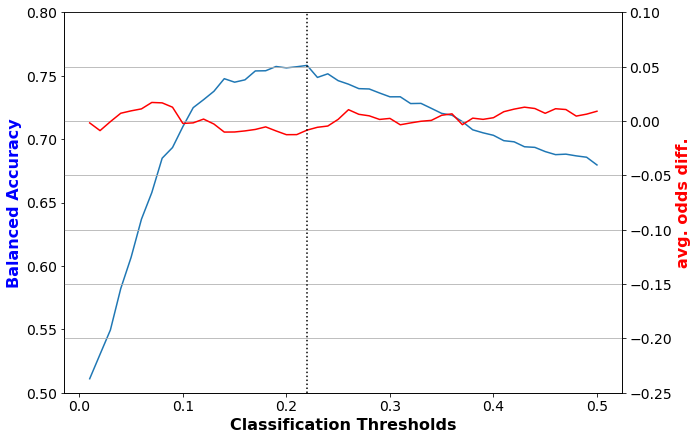In :
describe_metrics(val_metrics, thresh_arr)

Threshold corresponding to Best balanced accuracy: 0.2200
Best balanced accuracy: 0.7581
Corresponding 1-min(DI, 1/DI) value: 0.2939
Corresponding average odds difference value: -0.0084
Corresponding statistical parity difference value: -0.0992
Corresponding equal opportunity difference value: 0.0242
Corresponding Theil index value: 0.0938


#### 4.2.3. Testing LR model after reweighing¶

In :
lr_transf_metrics = test(dataset=dataset_orig_panel19_test,
model=lr_transf_panel19,
thresh_arr=[thresh_arr[lr_transf_best_ind]])

In :
describe_metrics(lr_transf_metrics, [thresh_arr[lr_transf_best_ind]])

Threshold corresponding to Best balanced accuracy: 0.2200
Best balanced accuracy: 0.7539
Corresponding 1-min(DI, 1/DI) value: 0.2482
Corresponding average odds difference value: -0.0151
Corresponding statistical parity difference value: -0.0872
Corresponding equal opportunity difference value: -0.0035
Corresponding Theil index value: 0.0966


The fairness metrics for the logistic regression model learned after reweighing are well improved, and thus the model is much more fair relative to the logistic regression model learned from the original data.

### 4.3. Learning a Random Forest (RF) classifier on data transformed by reweighing¶

#### 4.3.1. Training RF model after reweighing¶

In :
dataset = dataset_transf_panel19_train
model = make_pipeline(StandardScaler(),
RandomForestClassifier(n_estimators=500, min_samples_leaf=25))
fit_params = {'randomforestclassifier__sample_weight': dataset.instance_weights}
rf_transf_panel19 = model.fit(dataset.features, dataset.labels.ravel(), **fit_params)


#### 4.3.2. Validating RF model after reweighing¶

In :
thresh_arr = np.linspace(0.01, 0.5, 50)
val_metrics = test(dataset=dataset_orig_panel19_val,
model=rf_transf_panel19,
thresh_arr=thresh_arr)
rf_transf_best_ind = np.argmax(val_metrics['bal_acc'])

In :
disp_imp = np.array(val_metrics['disp_imp'])
disp_imp_err = 1 - np.minimum(disp_imp, 1/disp_imp)
plot(thresh_arr, 'Classification Thresholds',
val_metrics['bal_acc'], 'Balanced Accuracy',
disp_imp_err, '1 - min(DI, 1/DI)')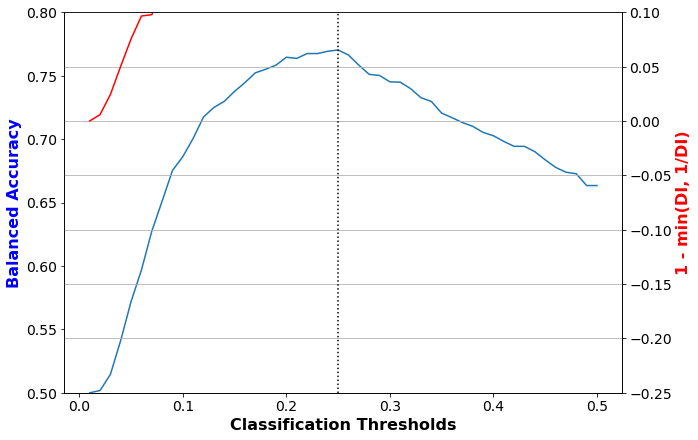In :
plot(thresh_arr, 'Classification Thresholds',
val_metrics['bal_acc'], 'Balanced Accuracy',
val_metrics['avg_odds_diff'], 'avg. odds diff.')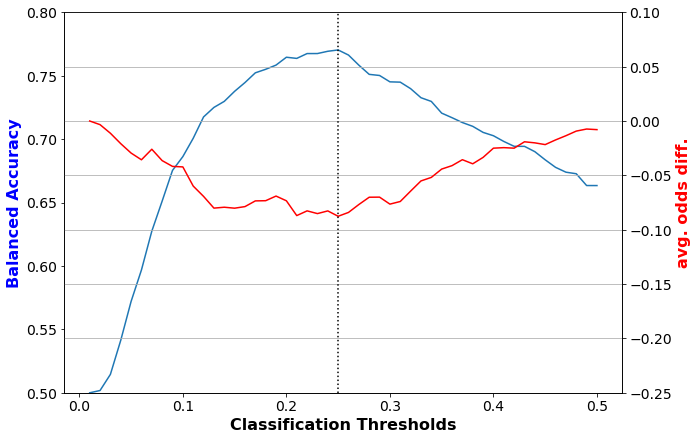In :
describe_metrics(val_metrics, thresh_arr)

Threshold corresponding to Best balanced accuracy: 0.2500
Best balanced accuracy: 0.7703
Corresponding 1-min(DI, 1/DI) value: 0.4516
Corresponding average odds difference value: -0.0876
Corresponding statistical parity difference value: -0.1668
Corresponding equal opportunity difference value: -0.0758
Corresponding Theil index value: 0.0906


#### 4.3.3. Testing RF model after reweighing¶

In :
rf_transf_metrics = test(dataset=dataset_orig_panel19_test,
model=rf_transf_panel19,
thresh_arr=[thresh_arr[rf_transf_best_ind]])

In :
describe_metrics(rf_transf_metrics, [thresh_arr[rf_transf_best_ind]])

Threshold corresponding to Best balanced accuracy: 0.2500
Best balanced accuracy: 0.7586
Corresponding 1-min(DI, 1/DI) value: 0.4307
Corresponding average odds difference value: -0.0843
Corresponding statistical parity difference value: -0.1632
Corresponding equal opportunity difference value: -0.0611
Corresponding Theil index value: 0.0963


Once again, the model learned from the transformed data is fairer than that learned from the original data. However, the random forest model learned from the transformed data is still relatively unfair as compared to the logistic regression model learned from the transformed data.

## 5. Bias mitigation using in-processing technique - Prejudice Remover (PR)¶

### 5.1. Learning a Prejudice Remover (PR) model on original data¶

#### 5.1.1. Training a PR model¶

In :
model = PrejudiceRemover(sensitive_attr=sens_attr, eta=25.0)
pr_orig_scaler = StandardScaler()

dataset = dataset_orig_panel19_train.copy()
dataset.features = pr_orig_scaler.fit_transform(dataset.features)

pr_orig_panel19 = model.fit(dataset)


#### 5.1.2. Validating PR model¶

In :
thresh_arr = np.linspace(0.01, 0.50, 50)

dataset = dataset_orig_panel19_val.copy()
dataset.features = pr_orig_scaler.transform(dataset.features)

val_metrics = test(dataset=dataset,
model=pr_orig_panel19,
thresh_arr=thresh_arr)
pr_orig_best_ind = np.argmax(val_metrics['bal_acc'])

In :
disp_imp = np.array(val_metrics['disp_imp'])
disp_imp_err = 1 - np.minimum(disp_imp, 1/disp_imp)
plot(thresh_arr, 'Classification Thresholds',
val_metrics['bal_acc'], 'Balanced Accuracy',
disp_imp_err, '1 - min(DI, 1/DI)')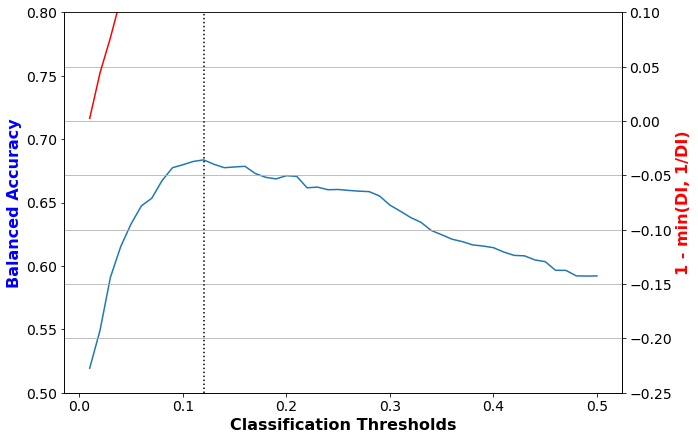In :
plot(thresh_arr, 'Classification Thresholds',
val_metrics['bal_acc'], 'Balanced Accuracy',
val_metrics['avg_odds_diff'], 'avg. odds diff.')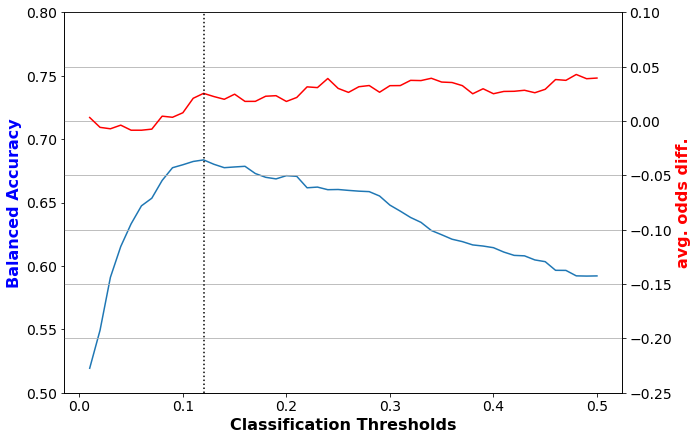In :
describe_metrics(val_metrics, thresh_arr)

Threshold corresponding to Best balanced accuracy: 0.1200
Best balanced accuracy: 0.6836
Corresponding 1-min(DI, 1/DI) value: 0.2268
Corresponding average odds difference value: 0.0254
Corresponding statistical parity difference value: -0.0830
Corresponding equal opportunity difference value: 0.1172
Corresponding Theil index value: 0.1119


#### 5.1.3. Testing PR model¶

In :
dataset = dataset_orig_panel19_test.copy()
dataset.features = pr_orig_scaler.transform(dataset.features)

pr_orig_metrics = test(dataset=dataset,
model=pr_orig_panel19,
thresh_arr=[thresh_arr[pr_orig_best_ind]])

In :
describe_metrics(pr_orig_metrics, [thresh_arr[pr_orig_best_ind]])

Threshold corresponding to Best balanced accuracy: 0.1200
Best balanced accuracy: 0.6880
Corresponding 1-min(DI, 1/DI) value: 0.1588
Corresponding average odds difference value: 0.0523
Corresponding statistical parity difference value: -0.0566
Corresponding equal opportunity difference value: 0.1479
Corresponding Theil index value: 0.1108


As in the case of reweighing, prejudice remover results in a fair model. However, it has come at the expense of relatively lower balanced accuracy.

## 6. Summary of Model Learning Results¶

In :
import pandas as pd
pd.set_option('display.multi_sparse', False)
results = [lr_orig_metrics, rf_orig_metrics, lr_transf_metrics,
rf_transf_metrics, pr_orig_metrics]
debias = pd.Series(['']*2 + ['Reweighing']*2
+ ['Prejudice Remover'],
name='Bias Mitigator')
clf = pd.Series(['Logistic Regression', 'Random Forest']*2 + [''],
name='Classifier')
pd.concat([pd.DataFrame(metrics) for metrics in results], axis=0).set_index([debias, clf])

Out:
avg_odds_diff bal_acc disp_imp eq_opp_diff stat_par_diff theil_ind
Bias Mitigator Classifier
Logistic Regression -0.205706 0.775935 0.426176 -0.222779 -0.261207 0.092122
Random Forest -0.138763 0.763772 0.485869 -0.113503 -0.218998 0.093575
Reweighing Logistic Regression -0.015104 0.753893 0.751755 -0.003518 -0.087196 0.096575
Reweighing Random Forest -0.084303 0.758565 0.569260 -0.061108 -0.163191 0.096345
Prejudice Remover 0.052286 0.688028 0.841229 0.147869 -0.056631 0.110774

Of all the models, the logistic regression model gives the best balance in terms of balanced accuracy and fairness. While the model learnt by prejudice remover is slightly fairer, it has much lower accuracy. All other models are quite unfair compared to the logistic model. Hence, we take the logistic regression model learnt from data transformed by re-weighing and 'deploy' it.

## 7. Deploying model¶

### 7.1. Testing model learned on 2014 (Panel 19) on 2015 (Panel 20) deployment data¶

In :
dataset_orig_panel20_deploy = MEPSDataset20()

# now align it with the 2014 dataset
dataset_orig_panel20_deploy = dataset_orig_panel19_train.align_datasets(dataset_orig_panel20_deploy)

In :
# describe(dataset_orig_panel20_train, dataset_orig_panel20_val, dataset_orig_panel20_deploy)
describe(test=dataset_orig_panel20_deploy)


#### Test Dataset shape¶

(17570, 138)


#### Favorable and unfavorable labels¶

1.0 0.0


#### Protected attribute names¶

['RACE']


#### Privileged and unprivileged protected attribute values¶

[array([1.])] [array([0.])]


#### Dataset feature names¶

['AGE', 'RACE', 'PCS42', 'MCS42', 'K6SUM42', 'REGION=1', 'REGION=2', 'REGION=3', 'REGION=4', 'SEX=1', 'SEX=2', 'MARRY=1', 'MARRY=2', 'MARRY=3', 'MARRY=4', 'MARRY=5', 'MARRY=6', 'MARRY=7', 'MARRY=8', 'MARRY=9', 'MARRY=10', 'FTSTU=-1', 'FTSTU=1', 'FTSTU=2', 'FTSTU=3', 'ACTDTY=1', 'ACTDTY=2', 'ACTDTY=3', 'ACTDTY=4', 'HONRDC=1', 'HONRDC=2', 'HONRDC=3', 'HONRDC=4', 'RTHLTH=-1', 'RTHLTH=1', 'RTHLTH=2', 'RTHLTH=3', 'RTHLTH=4', 'RTHLTH=5', 'MNHLTH=-1', 'MNHLTH=1', 'MNHLTH=2', 'MNHLTH=3', 'MNHLTH=4', 'MNHLTH=5', 'HIBPDX=-1', 'HIBPDX=1', 'HIBPDX=2', 'CHDDX=-1', 'CHDDX=1', 'CHDDX=2', 'ANGIDX=-1', 'ANGIDX=1', 'ANGIDX=2', 'MIDX=-1', 'MIDX=1', 'MIDX=2', 'OHRTDX=-1', 'OHRTDX=1', 'OHRTDX=2', 'STRKDX=-1', 'STRKDX=1', 'STRKDX=2', 'EMPHDX=-1', 'EMPHDX=1', 'EMPHDX=2', 'CHBRON=-1', 'CHBRON=1', 'CHBRON=2', 'CHOLDX=-1', 'CHOLDX=1', 'CHOLDX=2', 'CANCERDX=-1', 'CANCERDX=1', 'CANCERDX=2', 'DIABDX=-1', 'DIABDX=1', 'DIABDX=2', 'JTPAIN=-1', 'JTPAIN=1', 'JTPAIN=2', 'ARTHDX=-1', 'ARTHDX=1', 'ARTHDX=2', 'ARTHTYPE=-1', 'ARTHTYPE=1', 'ARTHTYPE=2', 'ARTHTYPE=3', 'ASTHDX=1', 'ASTHDX=2', 'ADHDADDX=-1', 'ADHDADDX=1', 'ADHDADDX=2', 'PREGNT=-1', 'PREGNT=1', 'PREGNT=2', 'WLKLIM=-1', 'WLKLIM=1', 'WLKLIM=2', 'ACTLIM=-1', 'ACTLIM=1', 'ACTLIM=2', 'SOCLIM=-1', 'SOCLIM=1', 'SOCLIM=2', 'COGLIM=-1', 'COGLIM=1', 'COGLIM=2', 'DFHEAR42=-1', 'DFHEAR42=1', 'DFHEAR42=2', 'DFSEE42=-1', 'DFSEE42=1', 'DFSEE42=2', 'ADSMOK42=-1', 'ADSMOK42=1', 'ADSMOK42=2', 'PHQ242=-1', 'PHQ242=0', 'PHQ242=1', 'PHQ242=2', 'PHQ242=3', 'PHQ242=4', 'PHQ242=5', 'PHQ242=6', 'EMPST=-1', 'EMPST=1', 'EMPST=2', 'EMPST=3', 'EMPST=4', 'POVCAT=1', 'POVCAT=2', 'POVCAT=3', 'POVCAT=4', 'POVCAT=5', 'INSCOV=1', 'INSCOV=2', 'INSCOV=3']

In :
metric_orig_panel20_deploy = BinaryLabelDatasetMetric(
dataset_orig_panel20_deploy,
unprivileged_groups=unprivileged_groups,
privileged_groups=privileged_groups)
explainer_orig_panel20_deploy = MetricTextExplainer(metric_orig_panel20_deploy)

print(explainer_orig_panel20_deploy.disparate_impact())

Disparate impact (probability of favorable outcome for unprivileged instances / probability of favorable outcome for privileged instances): 0.5456992351196291

In :
lr_transf_metrics_panel20_deploy = test(
dataset=dataset_orig_panel20_deploy,
model=lr_transf_panel19,
thresh_arr=[thresh_arr[lr_transf_best_ind]])

In :
describe_metrics(lr_transf_metrics_panel20_deploy, [thresh_arr[lr_transf_best_ind]])

Threshold corresponding to Best balanced accuracy: 0.2200
Best balanced accuracy: 0.7311
Corresponding 1-min(DI, 1/DI) value: 0.1943
Corresponding average odds difference value: 0.0071
Corresponding statistical parity difference value: -0.0596
Corresponding equal opportunity difference value: 0.0303
Corresponding Theil index value: 0.1019


Deployed model tested on the 2015 Panel 20 data still exhibits fairness as well as maintains accuracy.

## 8. Generating explanations for model predictions using LIME¶

### 8.1. Generating explanations on 2015 Panel 20 deployment data¶

This section shows how LIME can be integrated with AIF360 to get explanations for model predictions.

In :
train_dataset = dataset_transf_panel19_train  # data the deployed model (lr from transformed data)
test_dataset = dataset_orig_panel20_deploy  # the data model is being tested on
model = lr_transf_panel19  # lr_transf_panel19 is LR model learned from Panel 19 with Reweighing
thresh_arr = np.linspace(0.01, 0.5, 50)
best_thresh = thresh_arr[lr_transf_best_ind]


First, we need to fit the encoder to the aif360 dataset

In :
lime_data = LimeEncoder().fit(train_dataset)


The transform() method is then used to convert aif360 features to LIME-compatible features

In :
s_train = lime_data.transform(train_dataset.features)
s_test = lime_data.transform(test_dataset.features)


The LimeTabularExplainer takes as input the LIME-compatible data along with various other arguments to create a lime explainer

In :
explainer = LimeTabularExplainer(
s_train, class_names=lime_data.s_class_names,
feature_names=lime_data.s_feature_names,
categorical_features=lime_data.s_categorical_features,
categorical_names=lime_data.s_categorical_names,
kernel_width=3, verbose=False, discretize_continuous=True)


The inverse_transform() function is used to transform LIME-compatible data back to aif360-compatible data since that is needed by the model to make predictions. The function below is used to produce the predictions for any perturbed data that is produce by LIME

In :
def s_predict_fn(x):
return model.predict_proba(lime_data.inverse_transform(x))


The explain_instance() method can then be used to produce explanations for any instance in the test dataset

In :
def show_explanation(ind):
exp = explainer.explain_instance(s_test[ind], s_predict_fn, num_features=10)
exp.show_in_notebook(show_all=False)
print("Actual label: " + str(test_dataset.labels[ind]))

In :
print("Threshold corresponding to Best balanced accuracy: {:6.4f}".format(best_thresh))
show_explanation(0)
show_explanation(2)

Threshold corresponding to Best balanced accuracy: 0.2200